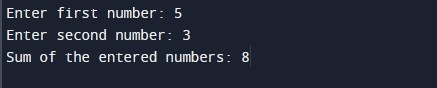# C Program to Add Two Numbers

In this tutorial you will learn about the C Program to Add Two Numbers and its application with practical example.

In this tutorial, we will learn to create a program to add two integer numbers using C programming language.

Prerequisites

Before starting with this tutorial we assume that you are best aware of the following C programming topics:

• C Data Types
• C Variables
• C Input Output
• C Operators

## Program to Add Two Numbers

In this program we will add two integer numbers entered by the user. We would first declared and initialized the required variables. Next, we would prompt user to input two integer numbers. Later in the program we will add the numbers and display the sum of the numbers.

Output:-In the above program, we have first declared and initialized a set variables required in the program.

• num1 = it holds the first integer number value
• num2 = it holds the second integer number value
• sum = it holds the sum of the number1 and number2

In the next statement user will be prompted to enter the two integer number values which will be assigned to variable ‘num1’ and ‘num2’ respectively. Next, we will perform addition of both integer numbers (num1 and num2) and assign result to sum. Now, we will be displaying the sum of two integer numbers using printf statement.

In this tutorial we have learn about the C Program to Add Two Numbers and its application with practical example. I hope you will like this tutorial.